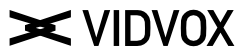# Vuo Accumulating MeasureTime

#1

I’m finding that many Vuo Time nodes require a Time Input that is consistently increasing.

How can I create this from the Measure Position time input which only goes from
0-1.000?

I’m ultimately looking for something to change the speed of the time that I’m inputing to a
Wave Node.

I’d like to be able to slow the Wave by .25 , 0.50 or speed by 1.5, 2, 2.5 etc

or should I be using the BPM and then applying that to the Time input?
would using the BPM to seconds NODE help?
IE 120 BPM would output 0.5 secs
Then if I divide the Time input by this number I would get 120 secs that would be elapsed over the 1 minute time.
time / bpmSeconds
60 / 0.5 = 120

For slowing down by 0.50 rate
I could either divide the BPM to seconds NODE Output by the rate:
bpmSeconds/rate
0.50 / 0.50 = 1
and then apply that?
time / ratedBpmSeconds
60 / 1 = 60 seconds

ratedBpmTime = time / (bpmSeconds / rate)

for 1/4 rate at 120 BPM

ratedBpmTime = 60 / (0.5 / 0.25)
rateBpmTime at 1Min = 30secs

thanks

0 Likes

#2

If you can’t find an answer for that here, I’d ask the Vuo community directly:
https://vuo.org/community/discussion

0 Likes